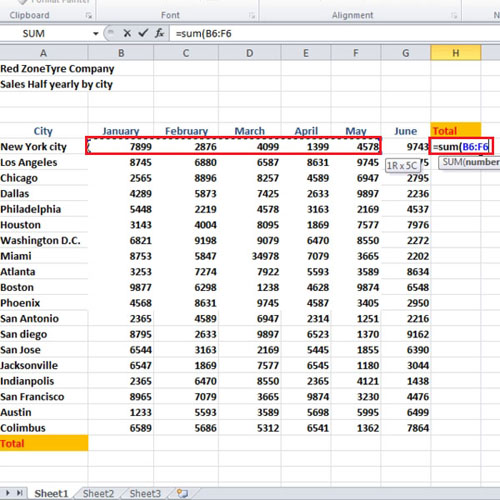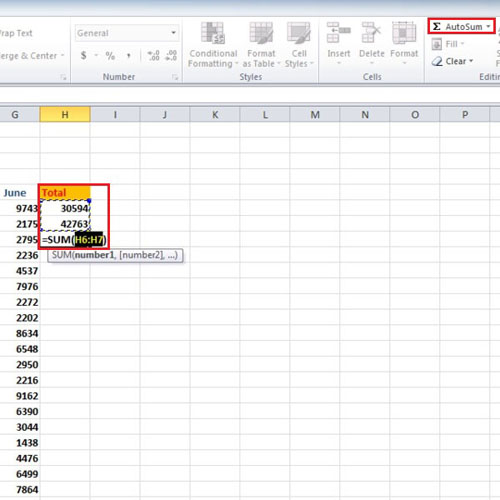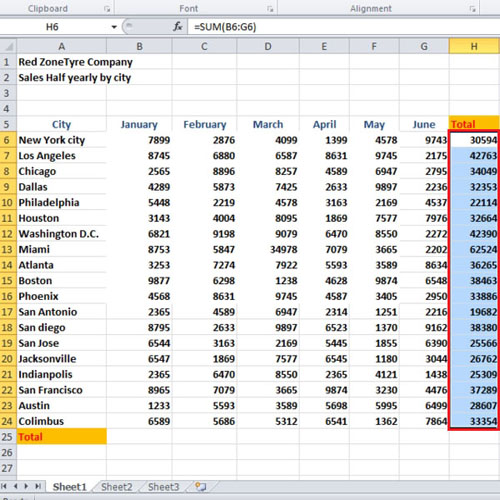## How to Using Sum and AutoSum in Excel 2010

Whenever you have to work with tons of numbers and make various calculations, use the sum function in Excel to make your task easy. You can also apply autosum in excel to make calculations for you. Furthermore in this tutorial, we will show you how to check these functions at a glance for any errors that might exist. Follow this step by step guide and master the sum functions that Excel has to offer.
In this tutorial, we will show you how to add numbers using Sum and AutoSum in Excel.

Step # 1 – Use the Sum function

Calculating the sum of many values can often be a complex task. You can use the sum function in excel to ease up your task. In order to do so, go to the cell where you want the total value to appear and type “=SUM( and select all the cells you want to add up and press the “enter” key.Step # 2 – Use the AutoSum function

Once done, go to the next cell and click on the “auto sum” button given under the “home” tab. Microsoft Excel will now automatically enter the formula here. In this manner, you can apply autosum in excel.Step # 3 – Check the functions

You can choose to manually select the rows you want to sum up and press the “ctrl” key and tilde to check the functions. Now go to column B to add them and use the “auto sum” function.